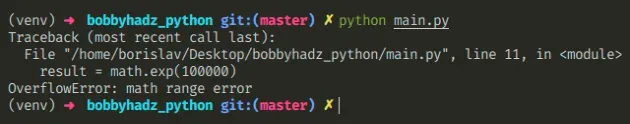# Solve - OverflowError: math range error in PythonSun May 01 20221 min readPhoto by Yarden

## Solve - OverflowError: math range error in Python#

The Python "OverflowError: math range error" occurs when the result of a mathematical calculation is too large. Use a `try/except` block to handle the error or use the `numpy` module if you have to manipulate larger numbers.Here is an example of how the error occurs.

main.py
```Copied!```import math

# ⛔️ OverflowError: math range error
result = math.exp(100000)
``````

The number we are trying to calculate is too large and it would take quite a while for the operation to complete, so an `OverflowError` is raised.

One way to handle the error is to use a `try/except` block.

main.py
```Copied!```import math

try:
result = math.exp(100000)
except OverflowError:
result = math.inf

print(result)  # 👉️ inf
``````

If calling the `math.exp()` method with the specified number raises an `OverflowError`, the `except` block is ran.

In the `except` block, we set the `result` variable to positive infinity.

The math.inf property returns a floating-point positive infinity.

It is equivalent to using `float('inf')`.

You can also set the variable to `None` or handle it in any other way that suits your use case.

An alternative approach is to install and use the `numpy` module.

Open your terminal in your project's root directory and install the `numpy` module.

shell
```Copied!```pip install numpy
``````

Now you can import and use the `numpy` module.

main.py
```Copied!```import numpy as np

result = np.exp(100000)

print(result)  # 👉️ inf
``````

You might get a runtime warning when running the code sample, but an error is not raised.

You can view the methods that are supported by the numpy package in the sidebar of the official docs.

Use the search field on my Home Page to filter through my more than 1,000 articles.